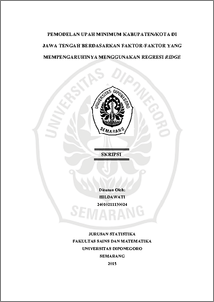# PEMODELAN UPAH MINIMUM KABUPATEN/KOTA DI JAWA TENGAH BERDASARKAN FAKTOR-FAKTOR YANG MEMPENGARUHINYA MENGGUNAKAN REGRESI RIDGE

HILDAWATI, HILDAWATI (2016) PEMODELAN UPAH MINIMUM KABUPATEN/KOTA DI JAWA TENGAH BERDASARKAN FAKTOR-FAKTOR YANG MEMPENGARUHINYA MENGGUNAKAN REGRESI RIDGE. Undergraduate thesis, FSM Universitas Diponegoro.Preview
PDF
3185Kb

## Abstract

The least squares method is a regression parameter estimation method for simple linear regression and multiple linear regression. This method produces no bias and variance estimator minimum if no multicollinearity. But if it happens, it will generate a large variance and covariance. One way to overcome this problem is by using ridge regression. Ridge regression is a modification of the least squares by adding a bias constant k^* on the main diagonal Z'Z. So that estimation parameter β ̂_R= (Z’Z+k^* I)^(-1) Z'Y^* with k^*≥0. This method produces bias and variance estimator minimum. Results of the modeling discussion of minimum wage in the city of Semarang, Surakarta, Tegal and Banyumas as well as the factors that influence it, such as inflation, Gross Domestic Regional Product (GDRP) and the Desent Living Needs contained multicollinearity problem. The minimum wage is significantly influenced Semarang Desent Living Needs, while Surakarta and Banyumas significantly affected GDRP and Desent Living Needs. Keywords: multicollinearity, ridge regression, bias constants k^*, the minimum wage

Item Type: Thesis (Undergraduate) H Social Sciences > HA Statistics Faculty of Science and Mathematics > Department of Statistics 49833 Mr Hasbi Yasin 23 Aug 2016 10:32 23 Aug 2016 10:32

Repository Staff Only: item control page